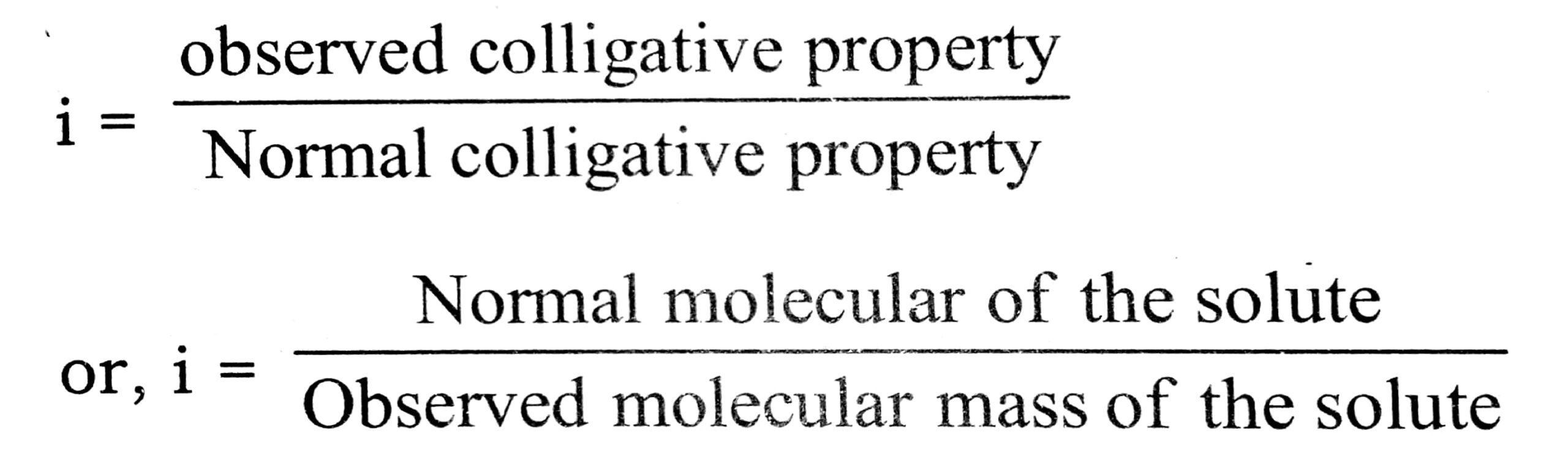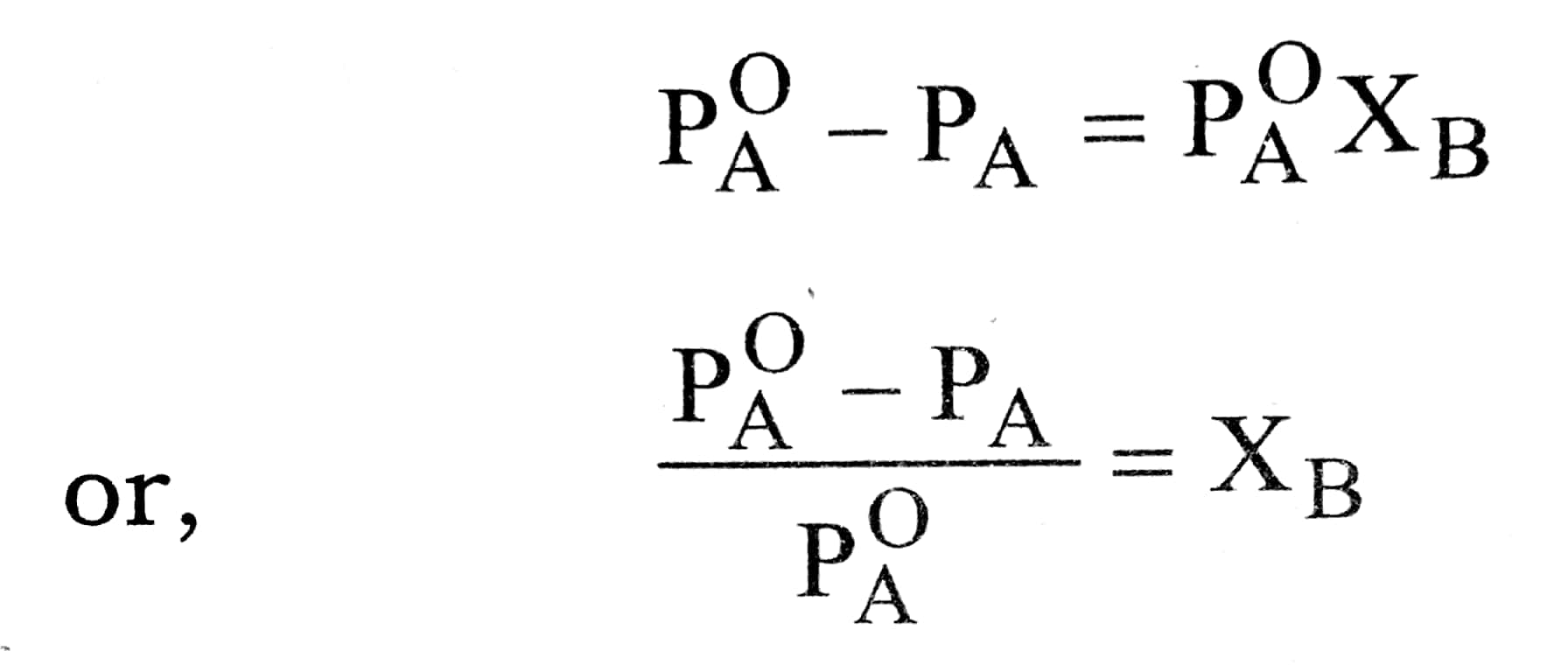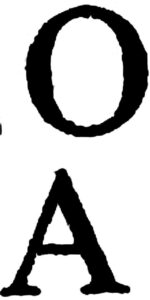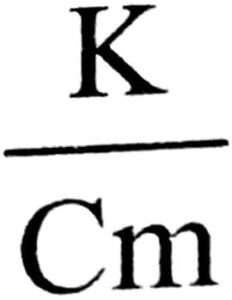2. SOLUTION

Q. 1. State the two characteristics of the super saturated solution.

Ans. There are two characteristics of the super saturated solution is :
(i) If a crystal of solute is added to super saturated solution, crystallisation occurs rapidly.
(ii) Super saturated solutions contain more solute than they should have at a particular temperature.

Q. 2. Which type of deviation is shown by the solution formed by mixing cyclohexane and ethanol ?

Ans. Hydrogen bonds exist among the molecules of ethanol (i.e., strong forces). When cyclohexane is added to ethanol, the cyclohexane molecule will come in between the ethanol molecules and disturb the hydrogen bondings. The forces between ethanol and cyclohexane will be weaker than the previous forces (H-bonding), hence there will be positive deviation.

Q. 3. What are ideal and non-ideal solutions ? Mention one nearly ideal solution.

Ans. The solution which obey Rault’s law and for which Hmix and Vmix are zero, are termed as ideal solutions.
The solutions which do not obey Raoult’s law are called non-ideal solutions.

Typical ideal solutions :
n-Hexane + n-Heptane
Ethyl bromide + Ethyl iodide
Benzene + Toluene

Q. 4. Methanol is soluble in water but insoluble in octane. Explain briefly.

Ans. Methanol and water both are polar and hydrogen bond forming molecules. Thus, they will form homogenous solution. Whereas octane is non-polar molecule and will not form solution with methanol.

Q. 5. What is the Van’t hoff factor ? How does is modify the equation for the elevation of boiling points ?

Ans. The Van’t Hoff factor is expressed asModified equation for the elevation of boiling point ΔTb = iKbm

Q. 6. Define the term solution. How inany types of solutions are formed ? Write briefly about each type with an example.

Ans. Solution : It is a homogeneous mixture of two or more components whose composition may be varied within limits. There are nine types of solutions.

Types of Solutions

 Solvent Solute Example Gas Gas Mixture of gases, air. Gas Liquid Water vapours in air, mist Gas Solid Sublimation of a solid into a gas: I2 vapours in nair Liquid Gas CO2 gas dissolved in water (aerated drinks like Limca) Liquid Liquid Mixture of miscible liquids,e.g., alcohol in water acetone in Water. Liquid Solid Sugar solution, salt solution in water. Solid Gas Adsorption of gases over metals; hydrogen over palladium. Solid Liquid Mercury in zinc, mercury in gold. Solid Solid Alloys, Brass etc.

Q. 7. State Raoult’s law. If ΔT is the elevation of boiling point of a solvent and m is the number of moles of solute per kg of solvent, what is the relationship between T and m?

Ans. Raoult’s law : It states that whenever a non volatile solue is added to a volatile solvent the vapour pressure of the solvent is lowered and the lowering of vapour pressures directly proportional to the mole fraction of the solute added.where, Pis the vapour pressure of the pure solvent.

PA is the vapour pressure of the solution.
XB is the mole fraction of non-volatile solute.
Thus, according to Raoult’s law, the relative lowering or vapour pressure is equal to the mole fraction of the solute in solution.

Q. 8. Define molar conductivity of an electrolytic solution. Mention the effect of the temperature on molar conductivity.

Ans. Molar conductivity is defined as electrolytic conductivity power of all the ions furnished by 1 mol of the electrolyte at a given concentration in solution. It is given as

^m =where ^m = Molar conductivity
K = Specific conductivity.
Cm = Concentration in molar per litre.
Effect of temperature on molar conductivity. The speed of movement of ions increases with an increase in temperature. Therefore, molar conductivity increases with temperature.

Q. 9. What is antifreze ?

Ans. A substance like ethylene glycol which is added to water to lower its freezing point.

Q. 10. Why NaCl solution freezes at lower temperature than water but boils at higher temperature than water ?

Ans. When a solute in dissolved in water, the vapour pressure decreases. Hence solution boils at a higher temperature, while solvent freezes at a lower temperature.

Q. 11. Two liquids X and Y boil at 110°C and 130°C respectively. Which one of them has higher vapour pressure at 50°C

Ans. Lower the B, Pt, more volatile the liquid. Hence X will have higher vapour pressure at 50°C.

Q. 12. Explain why non-ideal solution deviate from Raoult’s law ?

Ans. Non-ideal solutions deviate from Raoult’s law because the new forces of interaction between the components (A – B interactions) are different from those present in the pure components (A – A or B – B interactions).

Q. 13. Under what conditions van’t Hoff factor is (i) equal to one, (ii) greater than 1, (iii) less than 1.

Ans. (i) i = 1, when the solute does not undergo any association or dissociation in the solution.
(ii) i > 1, when the solute undergoes dissociation in the solution.
(111) i < 1, when the solute undergoes association in the solution.

Q. 14. Why an azeotropic mixture distils without any change in composition ?

Ans. Such a mixture on boiling forms vapours in the same mole fraction of the two components as present in the liquid mixture.

Q. 15. Henry’s law constant (KH) for He, O2 and N2 at 273 K are 6.71 x 10-6 bar-1, 4.44 × 10-5 bar-1 and 1.87 x 10-5 bar-1 respectively. What will be the order of their solubility in water at the same pressure and why ?

Ans. As KH are in bar-1, the Henry’s law will be in the form XA = KH PA. Thus, greater the value of KH greater is the solubility. Thus solubility will be in the order O2> N2 > He.

Q. 16. Why dissolution of some solid compounds in exothermic while that of some others in endothermic ?

Ans. If solute-solvent interactions are stronger than solute-solute or solvent-solvent interactions, energy is relased an the dissolution is exothermic. If solute-solvent intractions are weaker than solute-solute or solvent solvent intractions, energy is absorbed and the dissolution is endothermic.

Q. 17. When and why is molality preferred over molarity in handling solutions in chemistry ?

Ans. Molality is preferred when studies are made independent of temperature. This is because molality involves masses which do not change with temperature.

Q. 18. 10 cc of a liquid A where mixed with 10 cc of liquid B. The volume of the resulting solution was found to be 19.9 cc. What do you conclude ?

Ans. Decrease in volume means stronger intermolecular forces of attraction on mixing. This implies, that the solution shows negative deviation form Raoult’s law.

Q. 19. Two liquids A and B on mixing produce a warm solution. Which type of deviation from Roult’s law does it show ?

Ans. Warming up of the solution on mixing in solution indicates that the process is exothermic (ΔH mix is -ve). This implies that the solution shows negative deviation from Raoult’s law.

Q. 20. Which colligative property is preferred for the molar mass determination of macromolecules and why ?

Ans. Osmotic pressure determination is better suited for molar mass determination of macromolecules like proteins, polymers etc. Changes observed in other colligative properties for such solutions are usually very low and can’t be measured easily.

Q. 21. Sodium chloride or Calcium chloride is used to clear snow form the roads. Why ?

Ans. These salts depress the freezing point of water to such an extent that it cannot freeze to form ice. Hence, it melts off easily at the prevailing temperature.

Q. 22. What are colligative properties ? Mention them.

Ans. Colligative properties are those which depend upon the number of particles (atoms, molecules or atoms) of the solute and not on their nature. There are 4 colligative properties.

(i) Relative lowering of vapour pressure.
(ii) Elevation in Boiling point.
(iii) Depression in Freezing point.
(iv) Osmosis or osmotic pressure.

Q. 23. What happens when blood cells are placed in pure water ?

Ans. Due to osmosis, water molecules move into blood cells through the cell walls. As a result blood cells swell and may even burst.

Q. 24. What do you expect to happen when Red Blood Corpuscles (RBC’s) are placed in (i) 1% NaCl solution, (ii) 0.5% NaCl solution ?

Ans. (i) They will shrink due to plasmolysis.
(ii) They will swell and may even burst.
This is because RBC’s are isotonic with 0.91% NaCl Solution.

## Class 12th Chemistry Short Type Question English

 S.N CHEMISTRY SHORT TYPE QUESTION 2022 1 SOLID STATE 2 SOLUTION 3 ELECTROCHEMISTRY 4 CHEMICAL KINETICS 5 SURFACE CHEMISTRY 6 GENERAL PRINCIPLES AND PROCESSES OF ISOLATION OF ELEMENTS 7 THE P-BLOCK ELEMENTS 8 THE D- AND f-BLOCK ELEMENTS 9 CO-ORDINATION COMPOUNDS 10 HALOALKANES AND HALOARENES 11 ALCOHOLS, PHENOLS AND ETHERS 12 ALDEHYDES, KETONES AND CARBOXYLIC ACIDS 13 AMINES 14 BIOMOLECULES 15 POLYMERS 16 CHEMISTRY IN EVERYDAY LIFE